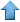Precision RecruitingSite Map
 [ Home ] [ Finance ] [ Web Audit ] [ Consulting ]

Statistical Software

1. Robust Multivariate Ridge and Linear Regression with Bootstrap

2. Stock Market Simulator

3. Simulation of Clustered Data

• Produces simulated clusters.
• Description: The seed routine creates a cluster of 1000 points, saved in cluster.txt: each row corresponds to a point; the first column is the cluster number, and the next two columns are the x and y coordinates. The cluster number is automatically incremented each time a new call to seed is made, resulting in the creation of a new cluster. The distance routine computes the distance between two points, for 100 points randomly selected in the data set previously created (cluster.txt). The output is a file dist.txt, with one row per pair of points, with two fields: the first column is an indicator and is equal to 1 if both points belong to the same cluster; the second column is the distance between the two points. This script illustrates how to check whether a data set contains one or two clusters by looking at the distribution of distances: a gap in the distribution means the presence of distinct clusters. It also suggests that the computational complexity of computing whether a data set contains one of more clusters is well below O(n), possibly O(n0.5), if one uses sampling techniques.
• Perl source code (77 lines)Data Mining • Machine Learning • Analytics • Quant • Statistics • Econometrics • Biostatistics • Web Analytics • Business Intelligence • Risk Management • Operations Research • AI • Predictive Modeling • Actuarial Sciences • Statistical Programming • Customer Insight • Data Modeling • Competitive Intelligence • Market Research • Information Retrieval • Computer Science • Retail Analytics • Healthcare Analytics • ROI Optimization • Design Of Experiments • Scoring Models • Six Sigma • SAS • Splus • SAP • ETL • SPSS • CRM • Cloud Computing • Electrical Engineering • Fraud Detection • Marketing Databases • Data Analysis • Decision Science • Text Mining